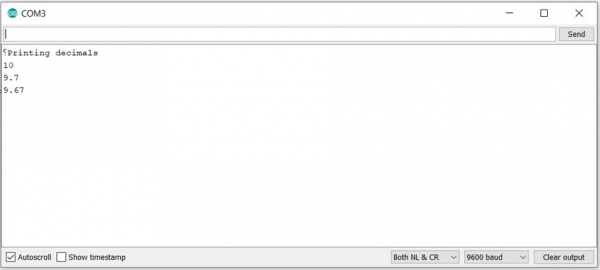# Reduce decimals while printing in Arduino

ArduinoArduino BoardsArduino IDEArduino Programming Language

Often some functions can output really long floating-point numbers, with several decimal digits. Several times, we are just interested in the first couple of decimal digits, and the remaining digits just reduce the readability and make the Serial Monitor window cluttered.

In order to round of floating-point numbers when printing to the Serial Monitor, you can just add the number of decimal places required as the second argument to serial.print.

An example is shown below −

## Example

void setup() {
// put your setup code here, to run once:
Serial.begin(9600);
Serial.println("Printing decimals");
Serial.println(9.6745,0); //This prints 10
Serial.println(9.6745,1); //This prints 9.7
Serial.println(9.6745,2); //This prints 9.67
}
void loop() {
// put your main code here, to run repeatedly:

}

The output of the above program on the Serial Monitor will be −

## OutputAs indicated in the comments, for the same floating-point number, the output will be different each time because we have specified different number of decimal places to use. Try it out yourself. Please note that this works only when your first argument to Serial.print() is a pure floating-point number. If it is a string, this won't work.

Thus, Serial.println("9.6745",0); won't print just '9'. In fact, this line will give you an error −

no matching function for call to 'println(const char , int)'